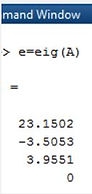naver-site-verification: naver47238a1b6bfbb19a2fd4b619734fa9a6.html
top of page

# Eigenvalues of a 4x4 matrix with Ti-84  (also complex)

## Explanation of calculating the eigen values of a matrix on your Ti84

You are visiting now the most popular page of ti84.org.

With the Ti84 Basic program EIGENVAL.8XP the eigenvalues of a matrix can be calculated (also complex). Useful for control system theory. For a 3*3 and 4*4 matrix, it is time-consuming if programs like Matlab or Maple are not available. With the program EIGENVAL.8xp, the eigenvalues up to a 4*4 matrix can be calculated.

2 examples are given: first, the eigenvalues of a 3*3 matrix is calculated. In the second examples, the eigen values of a 4*4 matrix are calculated.

Follow the next steps for calculating the eigenvalues (see the figures). It is advised to run the program Adefault.8xp first to get enough memory space.

1: make a 4*4 matrix [A] and fill the rows and the columns with the numbers. (Key 2nd Matrix-edit)

For a 3*3 or 2*2 matrix, still make a 4*4 matrix and fill the remaining places with 0.

2: Run the program EIGENVAL.8xp. The program calculates a4, a3, a2,a1,a0 of the equation: Solving det(A-λI)=0 results in :

### Resulting equationExample of a 3*3 Matrix:

Take a 4*4 matrix and fill the empty places with 0 in the 4*4 matrix for a 3* 3 problema4, a3, a2, a1, a0 are saved in List L1 and are ready to use in the app PlySmlt2

Run the Application PlySmlt2 and choose Poly Root Finder, degree 4 and after NEXT choose Load 2nd L1 and Solve. The eigenvalues are calculated.Eigenvalues

Comparison with Matlab shows that the program is correct. It is also tested on a 4x4 matrixFor questions or a request for the program EIGENVAL.8XP, mail to or chat.